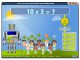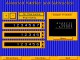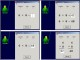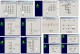Abilities Builder Math Facts 6.6 Learn whole number math facts addition, subtraction, multiplication, division. FreewareMath Games Multiplication 1.1 Practice multiplication (numbers 1 - 10) with the smart robot and his friends. FreewareYoungStar Skills Expert: Math 1.0 Out-of-the-box, the software provides 12 math skills activities including Number Basics, Addition Facts, Subtraction Facts, Addition or Subtraction, Counting by Multiples, Multiplication Facts, Division Facts, Quick Quads, Advanced Addition, Advanced Subtraction, Advanced Multiplication, and Advanced Division. DemoAbilities Builder Whole Number Math Facts 6.1 Learn whole number math facts: addition, subtraction, multiplication, division. DemoAnimated Arithmetic 1.0 Teaches addition, subtraction, multiplication and division for 1st - 4th grades. SharewareAbilities Builder Whole Number Math Facts 7.0 Learn whole number math facts: addition, subtraction, multiplication, division. DemoPanIntegers 2.0.1 PanIntegers is a software for the teaching and learning of integers including addition, subtraction, multiplication, division, order of calculations, removal of brackets and simple indices. SharewareInstant Interactive Drills 3.0 Instant Interactive Drills is part of the Instant Interactive Math Series product line. SharewareMeasure It 3.3 Whole number and fraction computation. Number by number problem exercises. DemoAdd & Subtract Fractions 3.3 Whole number and fraction computation. Number by number problem exercises. Demo